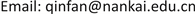﻿ 我国医药制造业产业集聚对创新能力的影响机制研究 The Impact of Pharmaceutical Industrial Agglomeration on Its Innovation Ability in China

World Economic Research
Vol. 07  No. 03 ( 2018 ), Article ID: 26776 , 8 pages
10.12677/WER.2018.73010

The Impact of Pharmaceutical Industrial Agglomeration on Its Innovation Ability in China

Fan Qin, Bing Fang, Yujin ZhuReceived: Aug. 16th, 2018; accepted: Sep. 4th, 2018; published: Sep. 11th, 2018ABSTRACT

Pharmaceutical industry is one of the six important high-tech industries in China. Improving its innovation ability is the key to enhance innovation ability and core competence of China. In the developing of pharmaceutical industry, the mechanism of the impact of pharmaceutical industrial agglomeration on its innovation ability is changing. This paper analyses the developing charactistics of Chinese pharmaceutical industry. By collecting and analyzing the complete data of 25 provinces and municipalities from 2010 to 2016, the impact of pharmaceutical industrial agglomeration status, competitive intensity, innovative labor and capital for innovation on innovation ability is discussed in this paper. Based on data analysis result, some strategies for improving Chinese pharmaceutical industry’s innovation ability are proposed.

Keywords:Spatial Econometrics, Industrial Agglomeration, Pharmaceutical Industry, Innovation Ability1. 引言

2. 文献回顾

3. 变量选择

3.1. 被解释变量

3.2. 解释变量

3.2.1. 专业化集聚水平(spei,j,t)

$sp{e}_{i,j,t}=\frac{{E}_{i,j,t}}{{E}_{i,t}}/\frac{{E}_{j,t}}{{E}_{t}}$

3.2.2. 多样化集聚水平(divi,j,t )

$di{v}_{i,j,t}=\frac{\frac{1}{{\sum }_{j\ne a}{\left(\frac{{E}_{i,a,t}}{{E}_{i,t}-{E}_{i,j,t}}\right)}^{2}}}{\frac{1}{{\sum }_{j\ne a}{\left(\frac{{E}_{a,t}}{{E}_{t}-{E}_{j,t}}\right)}^{2}}}=\frac{i\text{\hspace{0.17em}}地区\text{\hspace{0.17em}}j\text{\hspace{0.17em}}行业以外的其他行业\text{\hspace{0.17em}}t\text{\hspace{0.17em}}时期就业人口\text{\hspace{0.17em}}\text{HHI}\text{\hspace{0.17em}}的倒数}{所有样本地区\text{\hspace{0.17em}}j\text{\hspace{0.17em}}行业以外的其他行业\text{\hspace{0.17em}}t\text{\hspace{0.17em}}时期就业人口\text{\hspace{0.17em}}\text{HHI}\text{\hspace{0.17em}}的倒数}$

3.2.3. 市场竞争强度(comi,j,t )

$co{m}_{i,j,t}$ 表示i地区j行业t时期的市场竞争强度，由某个高技术产业在当地单位主营业务收入的企业数与该产业所有样本地区单位主营业务收入的企业数总和之间的比值来表示。该数值越大，说明相对于样本地区平均水平而言，该地区的市场竞争程度更强。市场竞争强度可表示为：

$co{m}_{i,j,t}=\frac{\frac{nu{m}_{i,j,t}}{in{c}_{i,j,t}}}{\frac{nu{m}_{j,t}}{in{c}_{j,t}}}$

3.2.4. 创新劳动力(Li,j,t )

Ｌ表示产业集群的科技研发人员投入比重，简称人员投入，及R & D人员全时当量占从业人员平均人数。可用R & D人员全时当量/从业人员平均人数来衡量  。

3.2.5. 创新资本(Ki,j,t )

3.3. 控制变量

3.3.1. 外商直接投资水平(fdii,t)

3.3.2. 人力资本水平(edui,t)

4. 模型选择

$\begin{array}{c}\mathrm{ln}I{P}_{i,j,t}^{k}={\rho }^{k}{w}_{i}^{\text{'}}\mathrm{ln}I{P}_{i,j,t}^{k}+\mathrm{ln}{x}_{i,j,t}^{k}\text{'}{\beta }^{k}\text{+}{w}_{i,j,t}^{\text{'}}\mathrm{ln}{x}_{i,j,t}^{k}\delta \\ +{\eta }_{i,j}^{k}+{\gamma }_{t}^{k}+{\epsilon }_{i,j,t}^{k}\end{array}$

${x}_{i,j,t}^{k}\text{'}$ 为解释变量及控制变量， $k\in \left\{\text{1},\text{2},\text{3},\text{4}\right\}$ ，表示不同的解释变量及控制变量集合。具体集合所包含的变量元素见表2。W为邻接空间矩阵。

5. 数据来源

6. 数据分析结果

7. 决策建议

1) 保持适当的产业专业化集聚水平和竞争强度，提高产业创新的专注力Table 1. Global Moran’s I of dependent variable

***为0.01水平下显著，**为0.05水平下显著，*为0.1水平下显著。

2) 增加创新资本投入，助推产业创新Table 4. Direct effects, indirect effects and total effects

***为0.01水平下显著，**为0.05水平下显著，*为0.1水平下显著。

3) 加强人才引进与本地人才培养，提升人才创新能力

8. 结论

The Impact of Pharmaceutical Industrial Agglomeration on Its Innovation Ability in China[J]. 世界经济探索, 2018, 07(03): 83-90. https://doi.org/10.12677/WER.2018.73010

1. 1. 徐菁. 兰州高新技术产业开发区现状、创新能力与产业集聚的实证分析[J]. 商场现代化, 2012(33): 131-133.

2. 2. 曾行, 李湘君, 王中华. 基于因子分析的江苏医药制造业创新能力评价[J]. 南京医科大学学报(社会科学版), 2018(1): 17-20.

3. 3. 吴其叶, 肖飞. 浙江省高新技术企业研发机构创新能力的空间集聚分析[J]. 科技管理研究, 2009(7): 207-210.

4. 4. 周明, 李宗植. 基于产业集聚的高技术产业创新能力研究[J]. 科研管理, 2011, 32(1): 15-21.

5. 5. 吴浩强, 刘树林. 并购对企业技术创新能力的影响——基于中国制造业分行业的视角[J]. 财会月刊, 2018(1): 130-134.

6. 6. 李勇, 许琳琳. 我国政府科技投入的技术创新产出效果实证分析——来自医药制造业的证据[J]. 铜陵学院学报, 2016(6): 8-12.

7. 7. 智越, 邱家学. 基于空间计量模型的医药制造业区域创新能力研究[J]. 上海医药, 2015(11): 54-56.

8. 8. 吕承超, 商圆月. 高技术产业集聚模式与创新产出的时空效应研究[J]. 管理科学, 2017(2): 64-79.

9. 9. 张舒淏. 专业化集聚与多样化集聚创新绩效比较研究[D]: [硕士学位论文]. 南京: 南京师范大学, 2015.

10. 10. Young, A. (2000) Gold Into Base Metals: Productivity Growth in the People’s Republic of China during the Reform Period. Nber Working Papers, 111, 1220-1261. https://doi.org/10.3386/w7856

11. 11. 张军, 吴桂英, 张吉鹏. 中国省际物质资本存量估算: 1952-2000 [J]. 经济研究, 2004(10): 35-44.

12. 12. 刘鹏, 张运峰. 产业集聚、FDI与城市创新能力——基于我国264个地级市数据的空间杜宾模型[J]. 华东经济管理, 2017(5): 56-65.

13. NOTES

1本文数据涉及的25个省及直辖市为：北京、天津、河北、山西、辽宁、吉林、黑龙江、上海、江苏、浙江、安徽、福建、江西、山东、河南、湖北、湖南、广东、广西、重庆、四川、贵州、云南、陕西、甘肃。